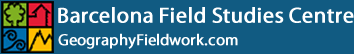•# Percentage or Divided Bar Graph Creator

This Percentage or Divided Bar Graph Creator converts raw data to percentages to create a bar graph to display the percentage of each subdivision. The length of each subdivision is proportional to the quantity it represents. The Divided Bar Graph option shows the frequency of each subdivision instead of percentage.

Enter your raw or percentage data in the calculator below. Click the 'Calculate' followed by 'Create Bar Graph' buttons and your bar chart will open in a new window. Hover over the subdivisions to display the data.

## Percentage or Divided Bar Graph Constructor

 Horizontal     Vertical    Divided    Percentage

Basic Bar Chart Creator (up to 100 categories)

Histogram or Bihistogram Creator